Successfully reported this slideshow.
We use your LinkedIn profile and activity data to personalize ads and to show you more relevant ads. You can change your ad preferences anytime.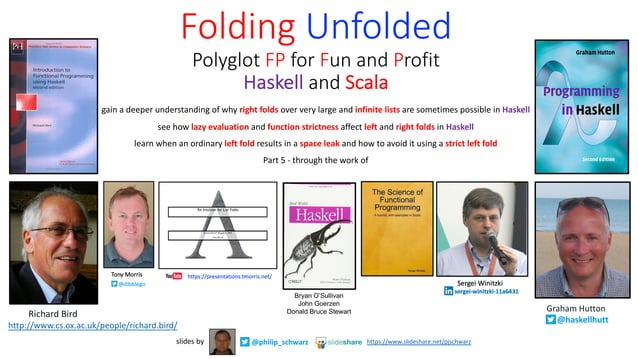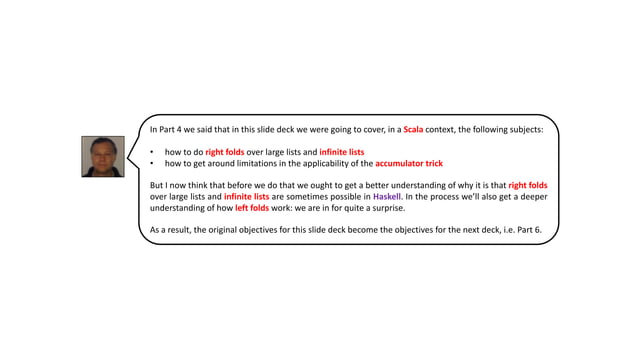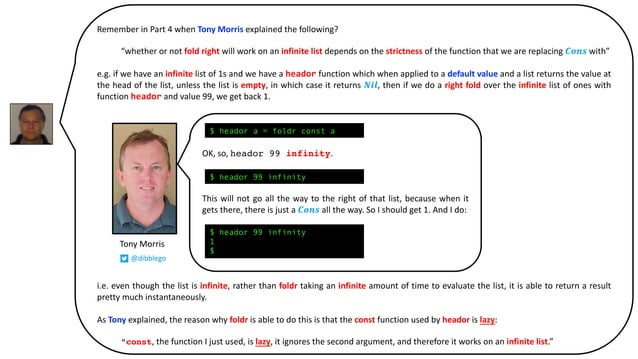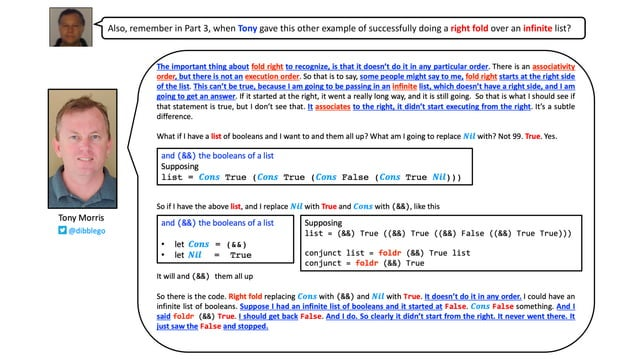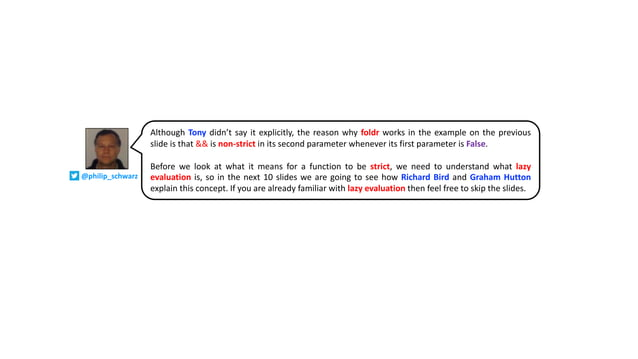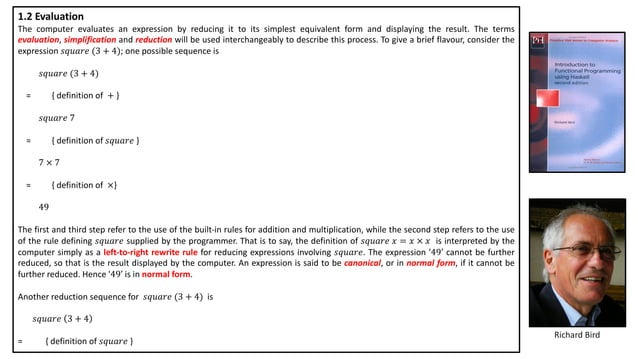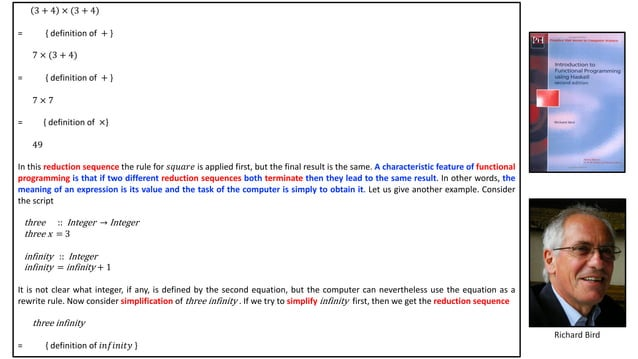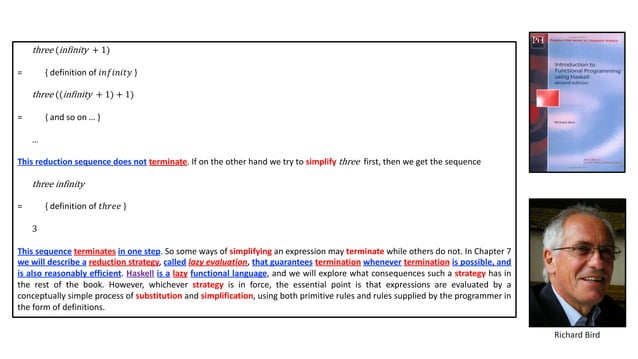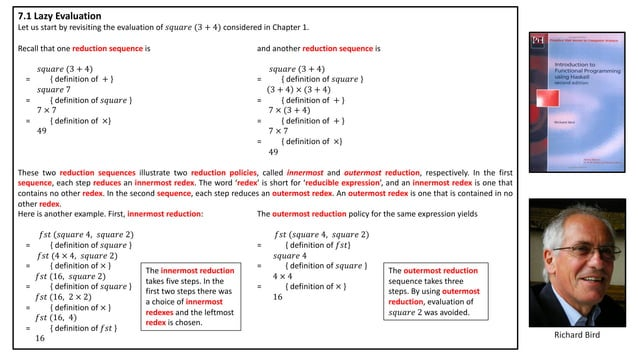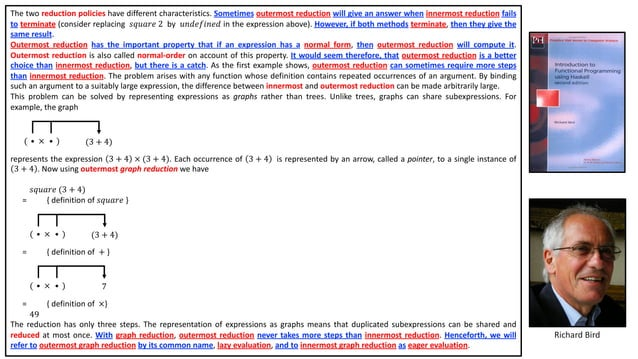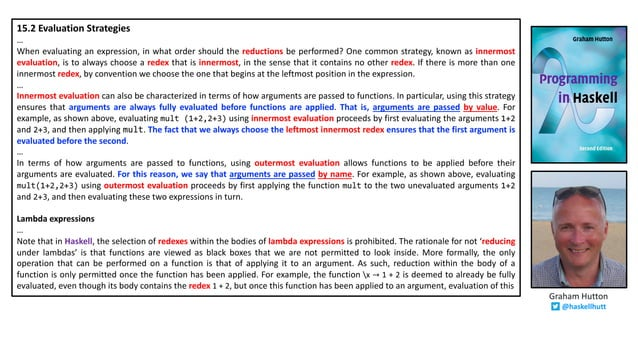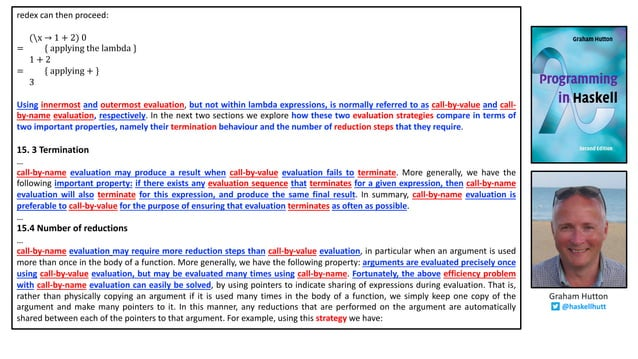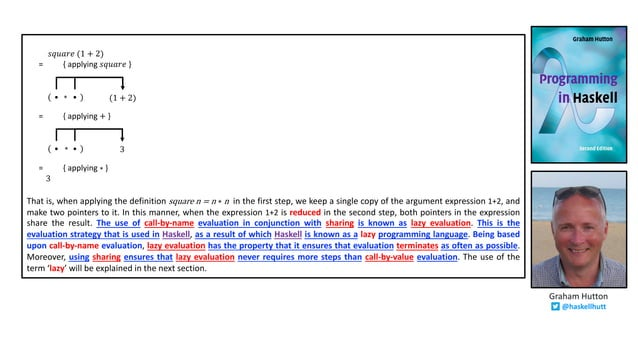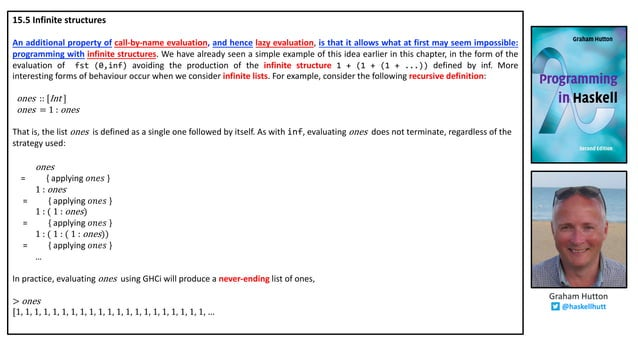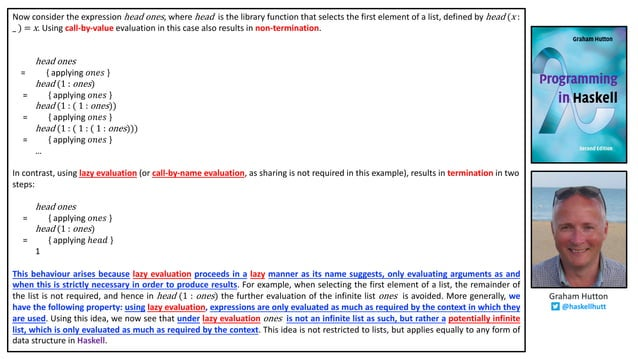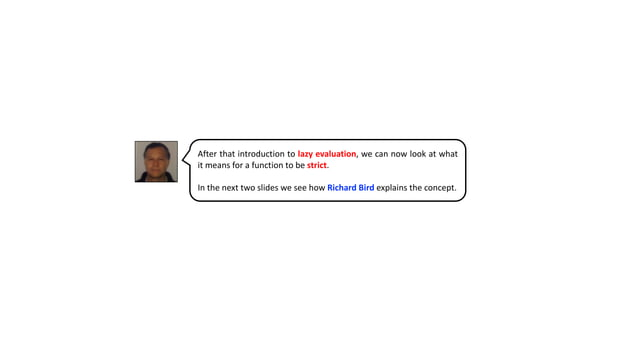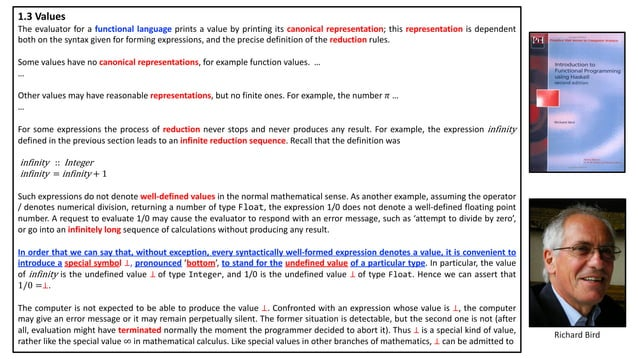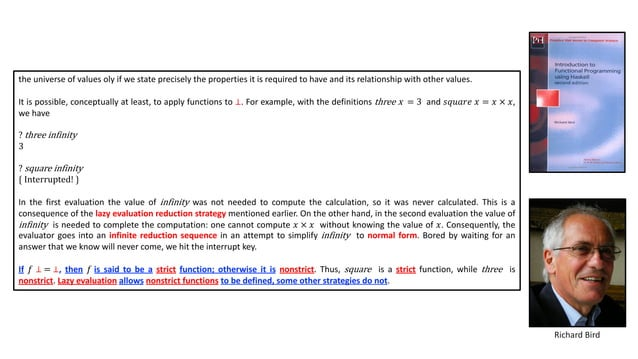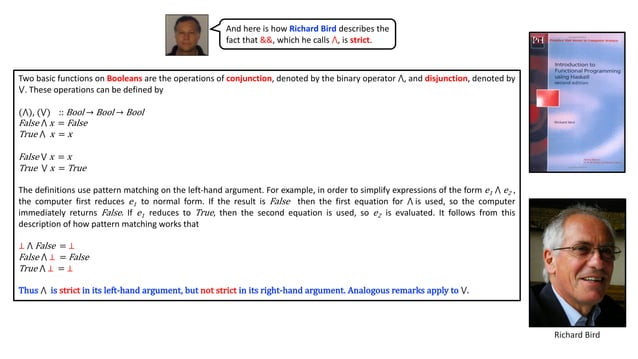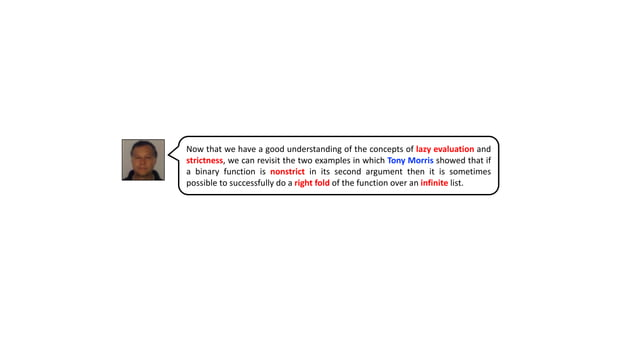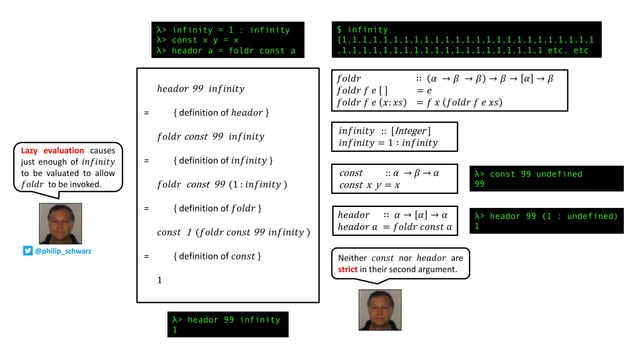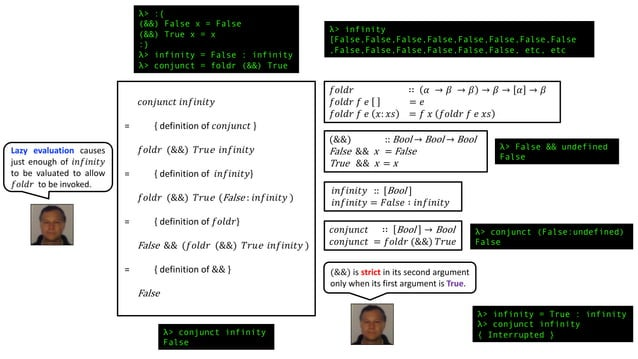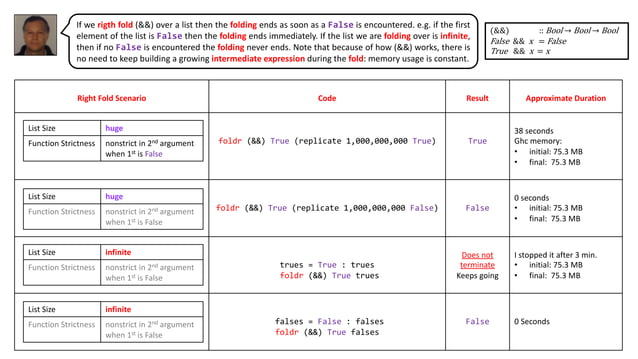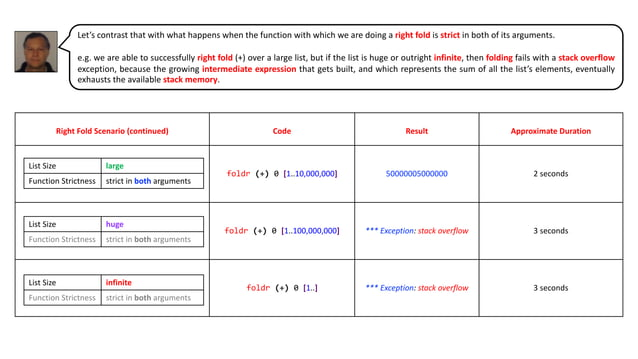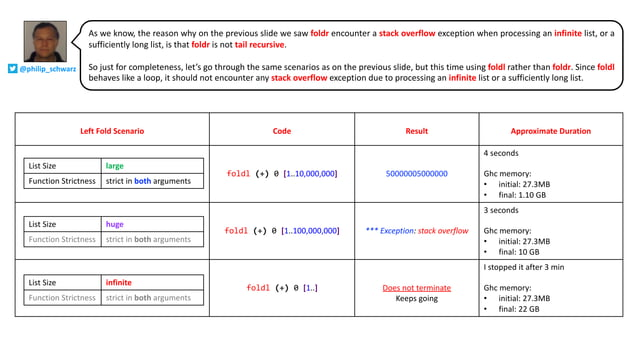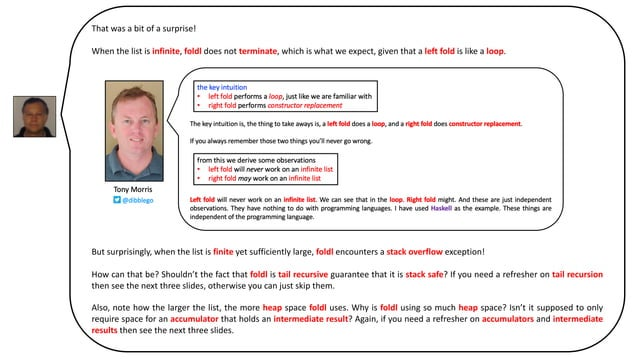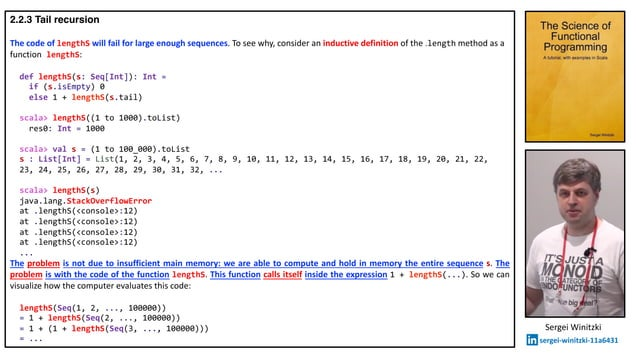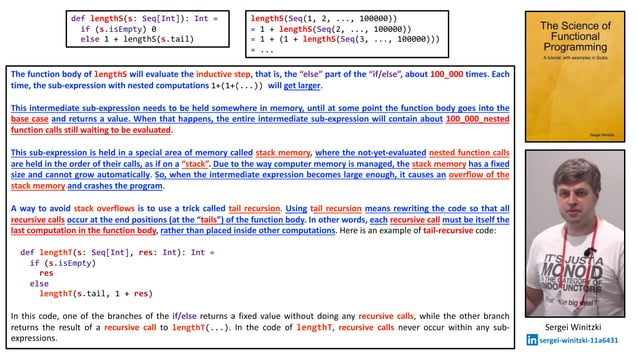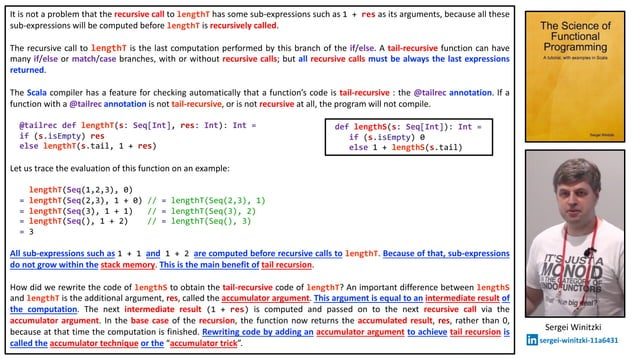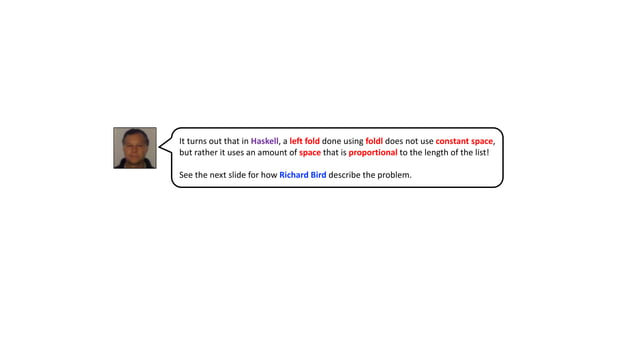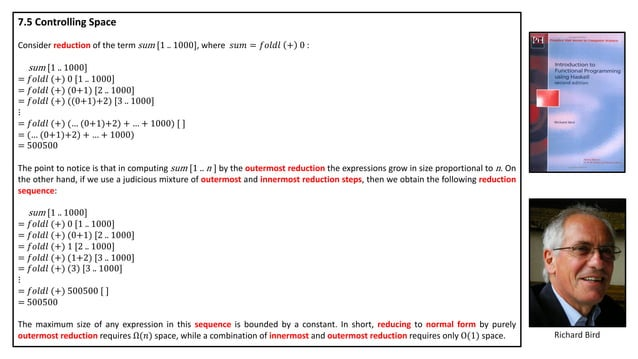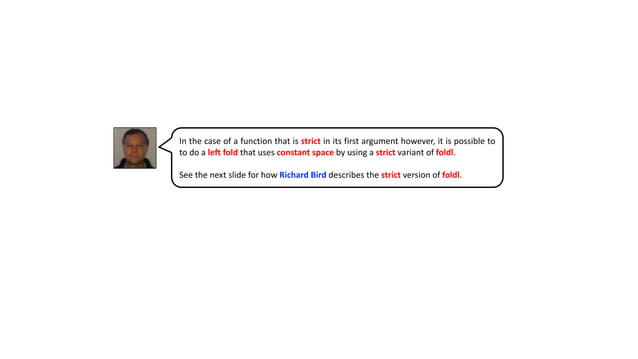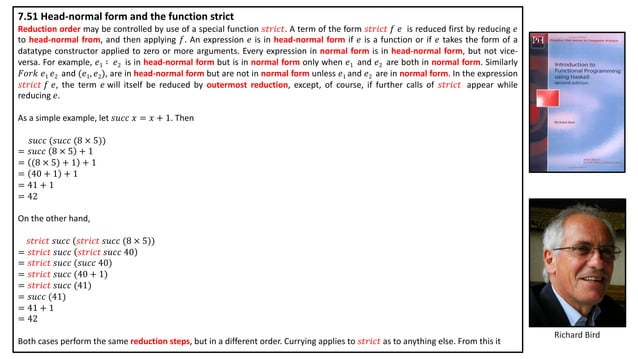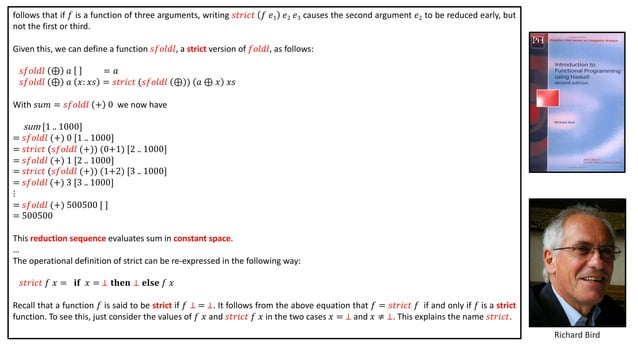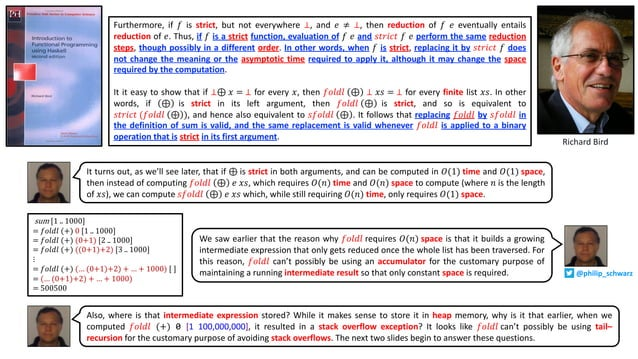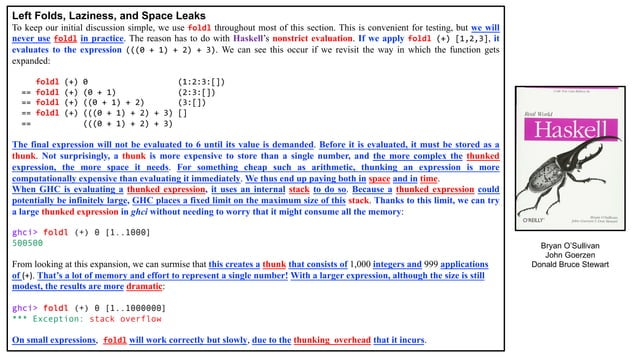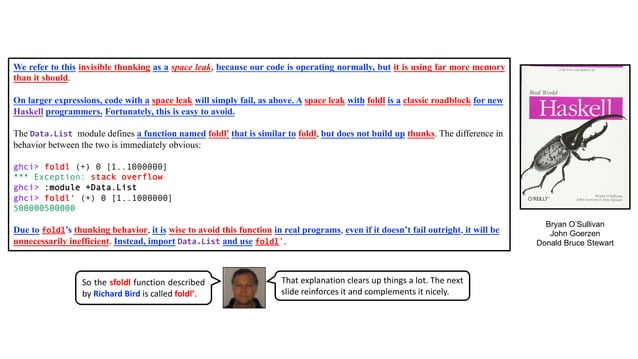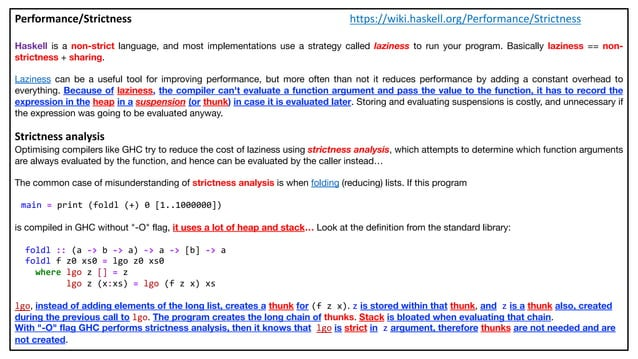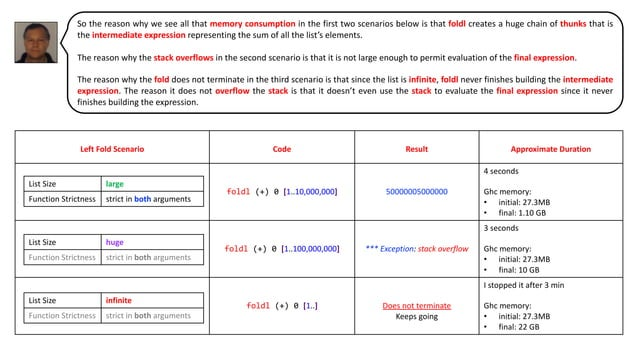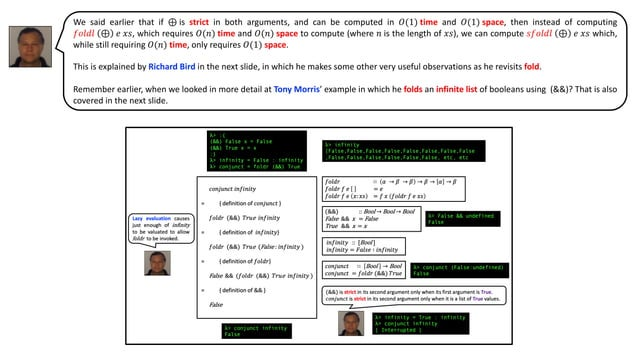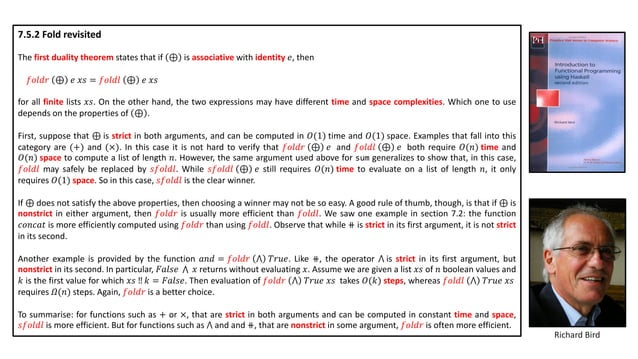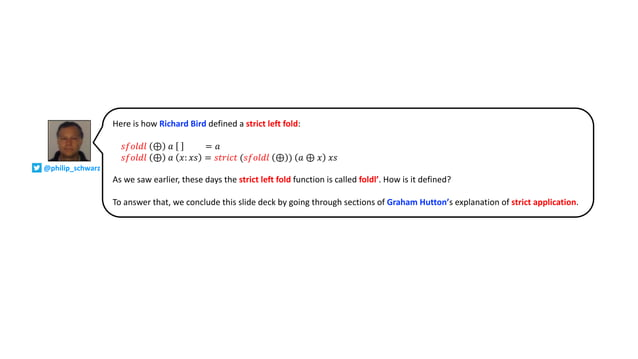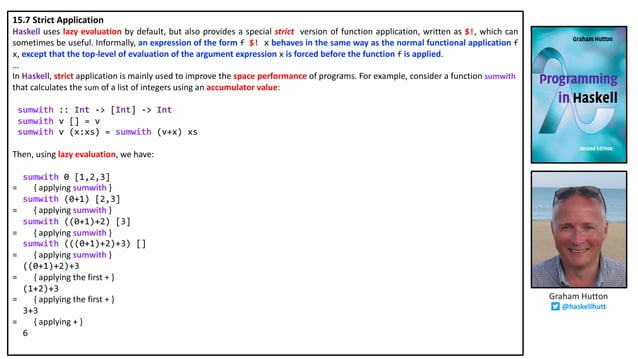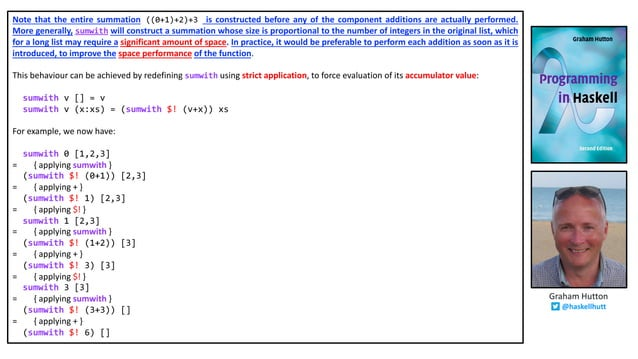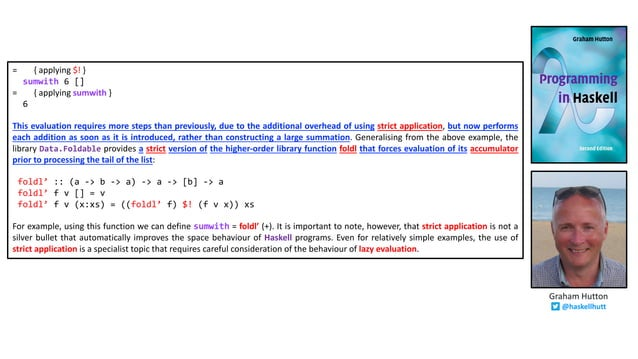Upcoming SlideShare
Loading in …5
×

# Folding Unfolded - Polyglot FP for Fun and Profit - Haskell and Scala - Part 5 - with minor corrections

(download for best quality slides) Gain a deeper understanding of why right folds over very large and infinite lists are sometimes possible in Haskell.
See how lazy evaluation and function strictness affect left and right folds in Haskell.
Learn when an ordinary left fold results in a space leak and how to avoid it using a strict left fold.

This version eliminates some minor imperfections and corrects the following two errors:
slide 15: "as sharing is required" should be "as sharing is not required"
slide 43: 푠푓표푙푑푙 (⊕) 푎 should be 푠푓표푙푑푙 (⊕) 푒

• Full Name
Comment goes here.

Are you sure you want to Yes No
Your message goes here• Be the first to comment

### Folding Unfolded - Polyglot FP for Fun and Profit - Haskell and Scala - Part 5 - with minor corrections

1. 1. gain a deeper understanding of why right folds over very large and infinite lists are sometimes possible in Haskell see how lazy evaluation and function strictness affect left and right folds in Haskell learn when an ordinary left fold results in a space leak and how to avoid it using a strict left fold Part 5 - through the work of Folding Unfolded Polyglot FP for Fun and Profit Haskell and Scala Richard Bird http://www.cs.ox.ac.uk/people/richard.bird/ Graham Hutton @haskellhutt Bryan O’Sullivan John Goerzen Donald Bruce Stewart @philip_schwarzslides by https://www.slideshare.net/pjschwarz
2. 2. In Part 4 we said that in this slide deck we were going to cover, in a Scala context, the following subjects: • how to do right folds over large lists and infinite lists • how to get around limitations in the applicability of the accumulator trick But I now think that before we do that we ought to get a better understanding of why it is that right folds over large lists and infinite lists are sometimes possible in Haskell. In the process we’ll also get a deeper understanding of how left folds work: we are in for quite a surprise. As a result, the original objectives for this slide deck become the objectives for the next deck, i.e. Part 6.
3. 3. Remember in Part 4 when Tony Morris explained the following? “whether or not fold right will work on an infinite list depends on the strictness of the function that we are replacing 𝑪𝒐𝒏𝒔 with” e.g. if we have an infinite list of 1s and we have a heador function which when applied to a default value and a list returns the value at the head of the list, unless the list is empty, in which case it returns 𝑵𝒊𝒍, then if we do a right fold over the infinite list of ones with function heador and value 99, we get back 1. i.e. even though the list is infinite, rather than foldr taking an infinite amount of time to evaluate the list, it is able to return a result pretty much instantaneously. As Tony explained, the reason why foldr is able to do this is that the const function used by heador is lazy: ”const, the function I just used, is lazy, it ignores the second argument, and therefore it works on an infinite list.” Tony Morris @dibblego OK, so, heador 99 infinity. This will not go all the way to the right of that list, because when it gets there, there is just a 𝑪𝒐𝒏𝒔 all the way. So I should get 1. And I do: \$ heador a = foldr const a \$ heador 99 infinity 1 \$ \$ heador 99 infinity
4. 4. Also, remember in Part 3, when Tony gave this other example of successfully doing a right fold over an infinite list?
5. 5. Although Tony didn’t say it explicitly, the reason why foldr works in the example on the previous slide is that && is non-strict in its second parameter whenever its first parameter is False. Before we look at what it means for a function to be strict, we need to understand what lazy evaluation is, so in the next 10 slides we are going to see how Richard Bird and Graham Hutton explain this concept. If you are already familiar with lazy evaluation then feel free to skip the slides. @philip_schwarz
6. 6. Richard Bird 1.2 Evaluation The computer evaluates an expression by reducing it to its simplest equivalent form and displaying the result. The terms evaluation, simplification and reduction will be used interchangeably to describe this process. To give a brief flavour, consider the expression 𝑠𝑞𝑢𝑎𝑟𝑒 (3 + 4); one possible sequence is 𝑠𝑞𝑢𝑎𝑟𝑒 (3 + 4) = { definition of + } 𝑠𝑞𝑢𝑎𝑟𝑒 7 = { definition of 𝑠𝑞𝑢𝑎𝑟𝑒 } 7 × 7 = { definition of ×} 49 The first and third step refer to the use of the built-in rules for addition and multiplication, while the second step refers to the use of the rule defining 𝑠𝑞𝑢𝑎𝑟𝑒 supplied by the programmer. That is to say, the definition of 𝑠𝑞𝑢𝑎𝑟𝑒 𝑥 = 𝑥 × 𝑥 is interpreted by the computer simply as a left-to-right rewrite rule for reducing expressions involving 𝑠𝑞𝑢𝑎𝑟𝑒. The expression ‘49’ cannot be further reduced, so that is the result displayed by the computer. An expression is said to be canonical, or in normal form, if it cannot be further reduced. Hence ‘49’ is in normal form. Another reduction sequence for 𝑠𝑞𝑢𝑎𝑟𝑒 (3 + 4) is 𝑠𝑞𝑢𝑎𝑟𝑒 3 + 4 = { definition of 𝑠𝑞𝑢𝑎𝑟𝑒 }
7. 7. Richard Bird 3 + 4 × (3 + 4) = { definition of + } 7 × (3 + 4) = { definition of + } 7 × 7 = { definition of ×} 49 In this reduction sequence the rule for 𝑠𝑞𝑢𝑎𝑟𝑒 is applied first, but the final result is the same. A characteristic feature of functional programming is that if two different reduction sequences both terminate then they lead to the same result. In other words, the meaning of an expression is its value and the task of the computer is simply to obtain it. Let us give another example. Consider the script three :: Integer → Integer three x = 3 infinity :: Integer infinity = infinity + 1 It is not clear what integer, if any, is defined by the second equation, but the computer can nevertheless use the equation as a rewrite rule. Now consider simplification of three infinity . If we try to simplify infinity first, then we get the reduction sequence three infinity = { definition of 𝑖𝑛𝑓𝑖𝑛𝑖𝑡𝑦 }
8. 8. three (infinity + 1) = { definition of 𝑖𝑛𝑓𝑖𝑛𝑖𝑡𝑦 } three ((infinity + 1) + 1) = { and so on … } … This reduction sequence does not terminate. If on the other hand we try to simplify three first, then we get the sequence three infinity = { definition of 𝑡ℎ𝑟𝑒𝑒 } 3 This sequence terminates in one step. So some ways of simplifying an expression may terminate while others do not. In Chapter 7 we will describe a reduction strategy, called lazy evaluation, that guarantees termination whenever termination is possible, and is also reasonably efficient. Haskell is a lazy functional language, and we will explore what consequences such a strategy has in the rest of the book. However, whichever strategy is in force, the essential point is that expressions are evaluated by a conceptually simple process of substitution and simplification, using both primitive rules and rules supplied by the programmer in the form of definitions. Richard Bird
9. 9. Richard Bird 7.1 Lazy Evaluation Let us start by revisiting the evaluation of 𝑠𝑞𝑢𝑎𝑟𝑒 (3 + 4) considered in Chapter 1. Recall that one reduction sequence is and another reduction sequence is 𝑠𝑞𝑢𝑎𝑟𝑒 (3 + 4) 𝑠𝑞𝑢𝑎𝑟𝑒 (3 + 4) = { definition of + } = { definition of 𝑠𝑞𝑢𝑎𝑟𝑒 } 𝑠𝑞𝑢𝑎𝑟𝑒 7 3 + 4 × (3 + 4) = { definition of 𝑠𝑞𝑢𝑎𝑟𝑒 } = { definition of + } 7 × 7 7 × (3 + 4) = { definition of ×} = { definition of + } 49 7 × 7 = { definition of ×} 49 These two reduction sequences illustrate two reduction policies, called innermost and outermost reduction, respectively. In the first sequence, each step reduces an innermost redex. The word ‘redex’ is short for ‘reducible expression’, and an innermost redex is one that contains no other redex. In the second sequence, each step reduces an outermost redex. An outermost redex is one that is contained in no other redex. Here is another example. First, innermost reduction: The outermost reduction policy for the same expression yields 𝑓𝑠𝑡 (𝑠𝑞𝑢𝑎𝑟𝑒 4, 𝑠𝑞𝑢𝑎𝑟𝑒 2) 𝑓𝑠𝑡 (𝑠𝑞𝑢𝑎𝑟𝑒 4, 𝑠𝑞𝑢𝑎𝑟𝑒 2) = { definition of 𝑠𝑞𝑢𝑎𝑟𝑒 } = { definition of 𝑓𝑠𝑡} 𝑓𝑠𝑡 (4 × 4, 𝑠𝑞𝑢𝑎𝑟𝑒 2) 𝑠𝑞𝑢𝑎𝑟𝑒 4 = { definition of × } = { definition of 𝑠𝑞𝑢𝑎𝑟𝑒 } 𝑓𝑠𝑡 (16, 𝑠𝑞𝑢𝑎𝑟𝑒 2) 4 × 4 = { definition of 𝑠𝑞𝑢𝑎𝑟𝑒 } = { definition of × } 𝑓𝑠𝑡 (16, 2 × 2) 16 = { definition of × } 𝑓𝑠𝑡 (16, 4) = { definition of 𝑓𝑠𝑡 } 16 The innermost reduction takes five steps. In the first two steps there was a choice of innermost redexes and the leftmost redex is chosen. The outermost reduction sequence takes three steps. By using outermost reduction, evaluation of 𝑠𝑞𝑢𝑎𝑟𝑒 2 was avoided.
10. 10. The two reduction policies have different characteristics. Sometimes outermost reduction will give an answer when innermost reduction fails to terminate (consider replacing 𝑠𝑞𝑢𝑎𝑟𝑒 2 by 𝑢𝑛𝑑𝑒𝑓𝑖𝑛𝑒𝑑 in the expression above). However, if both methods terminate, then they give the same result. Outermost reduction has the important property that if an expression has a normal form, then outermost reduction will compute it. Outermost reduction is also called normal-order on account of this property. It would seem therefore, that outermost reduction is a better choice than innermost reduction, but there is a catch. As the first example shows, outermost reduction can sometimes require more steps than innermost reduction. The problem arises with any function whose definition contains repeated occurrences of an argument. By binding such an argument to a suitably large expression, the difference between innermost and outermost reduction can be made arbitrarily large. This problem can be solved by representing expressions as graphs rather than trees. Unlike trees, graphs can share subexpressions. For example, the graph represents the expression 3 + 4 × (3 + 4). Each occurrence of 3 + 4 is represented by an arrow, called a pointer, to a single instance of 3 + 4 . Now using outermost graph reduction we have 𝑠𝑞𝑢𝑎𝑟𝑒 (3 + 4) = { definition of 𝑠𝑞𝑢𝑎𝑟𝑒 } = { definition of + } = { definition of ×} 49 The reduction has only three steps. The representation of expressions as graphs means that duplicated subexpressions can be shared and reduced at most once. With graph reduction, outermost reduction never takes more steps than innermost reduction. Henceforth, we will refer to outermost graph reduction by its common name, lazy evaluation, and to innermost graph reduction as eager evaluation. Richard Bird (3 + 4)• •( )× (3 + 4)• •( )× 7• •( )×
11. 11. 15.2 Evaluation Strategies … When evaluating an expression, in what order should the reductions be performed? One common strategy, known as innermost evaluation, is to always choose a redex that is innermost, in the sense that it contains no other redex. If there is more than one innermost redex, by convention we choose the one that begins at the leftmost position in the expression. … Innermost evaluation can also be characterized in terms of how arguments are passed to functions. In particular, using this strategy ensures that arguments are always fully evaluated before functions are applied. That is, arguments are passed by value. For example, as shown above, evaluating mult (1+2,2+3) using innermost evaluation proceeds by first evaluating the arguments 1+2 and 2+3, and then applying mult. The fact that we always choose the leftmost innermost redex ensures that the first argument is evaluated before the second. … In terms of how arguments are passed to functions, using outermost evaluation allows functions to be applied before their arguments are evaluated. For this reason, we say that arguments are passed by name. For example, as shown above, evaluating mult(1+2,2+3) using outermost evaluation proceeds by first applying the function mult to the two unevaluated arguments 1+2 and 2+3, and then evaluating these two expressions in turn. Lambda expressions … Note that in Haskell, the selection of redexes within the bodies of lambda expressions is prohibited. The rationale for not ‘reducing under lambdas’ is that functions are viewed as black boxes that we are not permitted to look inside. More formally, the only operation that can be performed on a function is that of applying it to an argument. As such, reduction within the body of a function is only permitted once the function has been applied. For example, the function x → 1 + 2 is deemed to already be fully evaluated, even though its body contains the redex 1 + 2, but once this function has been applied to an argument, evaluation of this Graham Hutton @haskellhutt
12. 12. redex can then proceed: (x → 1 + 2) 0 = { applying the lambda } 1 + 2 = { applying + } 3 Using innermost and outermost evaluation, but not within lambda expressions, is normally referred to as call-by-value and call- by-name evaluation, respectively. In the next two sections we explore how these two evaluation strategies compare in terms of two important properties, namely their termination behaviour and the number of reduction steps that they require. 15. 3 Termination … call-by-name evaluation may produce a result when call-by-value evaluation fails to terminate. More generally, we have the following important property: if there exists any evaluation sequence that terminates for a given expression, then call-by-name evaluation will also terminate for this expression, and produce the same final result. In summary, call-by-name evaluation is preferable to call-by-value for the purpose of ensuring that evaluation terminates as often as possible. … 15.4 Number of reductions … call-by-name evaluation may require more reduction steps than call-by-value evaluation, in particular when an argument is used more than once in the body of a function. More generally, we have the following property: arguments are evaluated precisely once using call-by-value evaluation, but may be evaluated many times using call-by-name. Fortunately, the above efficiency problem with call-by-name evaluation can easily be solved, by using pointers to indicate sharing of expressions during evaluation. That is, rather than physically copying an argument if it is used many times in the body of a function, we simply keep one copy of the argument and make many pointers to it. In this manner, any reductions that are performed on the argument are automatically shared between each of the pointers to that argument. For example, using this strategy we have: Graham Hutton @haskellhutt
13. 13. 𝑠𝑞𝑢𝑎𝑟𝑒 (1 + 2) = { applying 𝑠𝑞𝑢𝑎𝑟𝑒 } = { applying + } = { applying ∗ } 3 That is, when applying the definition square n = n ∗ n in the first step, we keep a single copy of the argument expression 1+2, and make two pointers to it. In this manner, when the expression 1+2 is reduced in the second step, both pointers in the expression share the result. The use of call-by-name evaluation in conjunction with sharing is known as lazy evaluation. This is the evaluation strategy that is used in Haskell, as a result of which Haskell is known as a lazy programming language. Being based upon call-by-name evaluation, lazy evaluation has the property that it ensures that evaluation terminates as often as possible. Moreover, using sharing ensures that lazy evaluation never requires more steps than call-by-value evaluation. The use of the term ‘lazy’ will be explained in the next section. (1 + 2)• •( )∗ 3• •( )∗ Graham Hutton @haskellhutt
14. 14. 15.5 Infinite structures An additional property of call-by-name evaluation, and hence lazy evaluation, is that it allows what at first may seem impossible: programming with infinite structures. We have already seen a simple example of this idea earlier in this chapter, in the form of the evaluation of fst (0,inf) avoiding the production of the infinite structure 1 + (1 + (1 + ...)) defined by inf. More interesting forms of behaviour occur when we consider infinite lists. For example, consider the following recursive definition: ones :: [Int ] ones = 1 : ones That is, the list ones is defined as a single one followed by itself. As with inf, evaluating ones does not terminate, regardless of the strategy used: ones = { applying 𝑜𝑛𝑒𝑠 } 1 : ones = { applying 𝑜𝑛𝑒𝑠 } 1 : ( 1 : ones) = { applying 𝑜𝑛𝑒𝑠 } 1 : ( 1 : ( 1 : ones)) = { applying 𝑜𝑛𝑒𝑠 } … In practice, evaluating ones using GHCi will produce a never-ending list of ones, > ones [1, 1, 1, 1, 1, 1, 1, 1, 1, 1, 1, 1, 1, 1, 1, 1, 1, 1, 1, 1, 1, … Graham Hutton @haskellhutt
15. 15. Now consider the expression head ones, where head is the library function that selects the first element of a list, defined by head (x : _ ) = x. Using call-by-value evaluation in this case also results in non-termination. head ones = { applying 𝑜𝑛𝑒𝑠 } head (1 : ones) = { applying 𝑜𝑛𝑒𝑠 } head (1 : ( 1 : ones)) = { applying 𝑜𝑛𝑒𝑠 } head (1 : ( 1 : ( 1 : ones))) = { applying 𝑜𝑛𝑒𝑠 } … In contrast, using lazy evaluation (or call-by-name evaluation, as sharing is not required in this example), results in termination in two steps: head ones = { applying 𝑜𝑛𝑒𝑠 } head (1 : ones) = { applying ℎ𝑒𝑎𝑑 } 1 This behaviour arises because lazy evaluation proceeds in a lazy manner as its name suggests, only evaluating arguments as and when this is strictly necessary in order to produce results. For example, when selecting the first element of a list, the remainder of the list is not required, and hence in head (1 : ones) the further evaluation of the infinite list ones is avoided. More generally, we have the following property: using lazy evaluation, expressions are only evaluated as much as required by the context in which they are used. Using this idea, we now see that under lazy evaluation ones is not an infinite list as such, but rather a potentially infinite list, which is only evaluated as much as required by the context. This idea is not restricted to lists, but applies equally to any form of data structure in Haskell. Graham Hutton @haskellhutt
16. 16. After that introduction to lazy evaluation, we can now look at what it means for a function to be strict. In the next two slides we see how Richard Bird explains the concept.
17. 17. Richard Bird 1.3 Values The evaluator for a functional language prints a value by printing its canonical representation; this representation is dependent both on the syntax given for forming expressions, and the precise definition of the reduction rules. Some values have no canonical representations, for example function values. … … Other values may have reasonable representations, but no finite ones. For example, the number 𝜋 … … For some expressions the process of reduction never stops and never produces any result. For example, the expression infinity defined in the previous section leads to an infinite reduction sequence. Recall that the definition was infinity :: Integer infinity = infinity + 1 Such expressions do not denote well-defined values in the normal mathematical sense. As another example, assuming the operator / denotes numerical division, returning a number of type Float, the expression 1/0 does not denote a well-defined floating point number. A request to evaluate 1/0 may cause the evaluator to respond with an error message, such as ‘attempt to divide by zero’, or go into an infinitely long sequence of calculations without producing any result. In order that we can say that, without exception, every syntactically well-formed expression denotes a value, it is convenient to introduce a special symbol ⊥, pronounced ’bottom’, to stand for the undefined value of a particular type. In particular, the value of infinity is the undefined value ⊥ of type Integer, and 1/0 is the undefined value ⊥ of type Float. Hence we can assert that 1/0 =⊥. The computer is not expected to be able to produce the value ⊥. Confronted with an expression whose value is ⊥, the computer may give an error message or it may remain perpetually silent. The former situation is detectable, but the second one is not (after all, evaluation might have terminated normally the moment the programmer decided to abort it). Thus ⊥ is a special kind of value, rather like the special value ∞ in mathematical calculus. Like special values in other branches of mathematics, ⊥ can be admitted to
18. 18. Richard Bird the universe of values oly if we state precisely the properties it is required to have and its relationship with other values. It is possible, conceptually at least, to apply functions to ⊥. For example, with the definitions three x = 3 and 𝑠𝑞𝑢𝑎𝑟𝑒 𝑥 = 𝑥 × 𝑥, we have ? three infinity 3 ? square infinity { Interrupted! } In the first evaluation the value of infinity was not needed to compute the calculation, so it was never calculated. This is a consequence of the lazy evaluation reduction strategy mentioned earlier. On the other hand, in the second evaluation the value of infinity is needed to complete the computation: one cannot compute 𝑥 × 𝑥 without knowing the value of 𝑥. Consequently, the evaluator goes into an infinite reduction sequence in an attempt to simplify infinity to normal form. Bored by waiting for an answer that we know will never come, we hit the interrupt key. If 𝑓 ⊥ = ⊥, then 𝑓 is said to be a strict function; otherwise it is nonstrict. Thus, square is a strict function, while three is nonstrict. Lazy evaluation allows nonstrict functions to be defined, some other strategies do not.
19. 19. And here is how Richard Bird describes the fact that &&, which he calls ⋀, is strict. Richard Bird Two basic functions on Booleans are the operations of conjunction, denoted by the binary operator ⋀, and disjunction, denoted by ⋁. These operations can be defined by (⋀), (⋁) :: Bool → Bool → Bool False ⋀ x = False True ⋀ x = x False ⋁ x = x True ⋁ x = True The definitions use pattern matching on the left-hand argument. For example, in order to simplify expressions of the form e1 ⋀ e2 , the computer first reduces e1 to normal form. If the result is False then the first equation for ⋀ is used, so the computer immediately returns False. If e1 reduces to True, then the second equation is used, so e2 is evaluated. It follows from this description of how pattern matching works that ⊥ ⋀ False = ⊥ False ⋀ ⊥ = False True ⋀ ⊥ = ⊥ Thus ⋀ is strict in its left-hand argument, but not strict in its right-hand argument. Analogous remarks apply to ⋁.
20. 20. Now that we have a good understanding of the concepts of lazy evaluation and strictness, we can revisit the two examples in which Tony Morris showed that if a binary function is nonstrict in its second argument then it is sometimes possible to successfully do a right fold of the function over an infinite list.
21. 21. ℎ𝑒𝑎𝑑𝑜𝑟 99 𝑖𝑛𝑓𝑖𝑛𝑖𝑡𝑦 = { definition of ℎ𝑒𝑎𝑑𝑜𝑟 } 𝑓𝑜𝑙𝑑𝑟 const 99 𝑖𝑛𝑓𝑖𝑛𝑖𝑡𝑦 = { definition of 𝑖𝑛𝑓𝑖𝑛𝑖𝑡𝑦 } 𝑓𝑜𝑙𝑑𝑟 const 99 (1 : 𝑖𝑛𝑓𝑖𝑛𝑖𝑡𝑦 ) = { definition of 𝑓𝑜𝑙𝑑𝑟 } 𝑐𝑜𝑛𝑠𝑡 1 (𝑓𝑜𝑙𝑑𝑟 𝑐𝑜𝑛𝑠𝑡 99 𝑖𝑛𝑓𝑖𝑛𝑖𝑡𝑦 ) = { definition of 𝑐𝑜𝑛𝑠𝑡 } 1 const :: 𝛼 → 𝛽 → 𝛼 const x y = x 𝑓𝑜𝑙𝑑𝑟 ∷ 𝛼 → 𝛽 → 𝛽 → 𝛽 → 𝛼 → 𝛽 𝑓𝑜𝑙𝑑𝑟 𝑓 𝑒 = 𝑒 𝑓𝑜𝑙𝑑𝑟 𝑓 𝑒 𝑥: 𝑥𝑠 = 𝑓 𝑥 𝑓𝑜𝑙𝑑𝑟 𝑓 𝑒 𝑥𝑠 ℎ𝑒𝑎𝑑𝑜𝑟 ∷ 𝛼 → 𝛼 → 𝛼 ℎ𝑒𝑎𝑑𝑜𝑟 𝑎 = 𝑓𝑜𝑙𝑑𝑟 𝑐𝑜𝑛𝑠𝑡 𝑎 𝑖𝑛𝑓𝑖𝑛𝑖𝑡𝑦 :: [Integer ] 𝑖𝑛𝑓𝑖𝑛𝑖𝑡𝑦 = 1 ∶ 𝑖𝑛𝑓𝑖𝑛𝑖𝑡𝑦 λ> infinity = 1 : infinity λ> const x y = x λ> heador a = foldr const a λ> heador 99 (1 : undefined) 1 λ> heador 99 infinity 1 λ> const 99 undefined 99 \$ infinity [1,1,1,1,1,1,1,1,1,1,1,1,1,1,1,1,1,1,1,1,1,1,1,1,1 ,1,1,1,1,1,1,1,1,1,1,1,1,1,1,1,1,1,1,1,1 etc, etc Lazy evaluation causes just enough of 𝑖𝑛𝑓𝑖𝑛𝑖𝑡𝑦 to be valuated to allow 𝑓𝑜𝑙𝑑𝑟 to be invoked. Neither 𝑐𝑜𝑛𝑠𝑡 nor ℎ𝑒𝑎𝑑𝑜𝑟 are strict in their second argument. @philip_schwarz
22. 22. 𝑐𝑜𝑛𝑗𝑢𝑛𝑐𝑡 ∷ Bool → Bool 𝑐𝑜𝑛𝑗𝑢𝑛𝑐𝑡 = 𝑓𝑜𝑙𝑑𝑟 (&&) 𝑇𝑟𝑢𝑒 𝑐𝑜𝑛𝑗𝑢𝑛𝑐𝑡 𝑖𝑛𝑓𝑖𝑛𝑖𝑡𝑦 = { definition of 𝑐𝑜𝑛𝑗𝑢𝑛𝑐𝑡 } 𝑓𝑜𝑙𝑑𝑟 (&&) 𝑇𝑟𝑢e 𝑖𝑛𝑓𝑖𝑛𝑖𝑡𝑦 = { definition of 𝑖𝑛𝑓𝑖𝑛𝑖𝑡𝑦} 𝑓𝑜𝑙𝑑𝑟 (&&) 𝑇𝑟𝑢e (False : 𝑖𝑛𝑓𝑖𝑛𝑖𝑡𝑦 ) = { definition of 𝑓𝑜𝑙𝑑𝑟} False && (𝑓𝑜𝑙𝑑𝑟 (&&) 𝑇𝑟𝑢e 𝑖𝑛𝑓𝑖𝑛𝑖𝑡𝑦 ) = { definition of && } False (&&) :: Bool → Bool → Bool False && x = False True && x = x λ> False && undefined False λ> :{ (&&) False x = False (&&) True x = x :} λ> infinity = False : infinity λ> conjunct = foldr (&&) True 𝑓𝑜𝑙𝑑𝑟 ∷ 𝛼 → 𝛽 → 𝛽 → 𝛽 → 𝛼 → 𝛽 𝑓𝑜𝑙𝑑𝑟 𝑓 𝑒 = 𝑒 𝑓𝑜𝑙𝑑𝑟 𝑓 𝑒 𝑥: 𝑥𝑠 = 𝑓 𝑥 𝑓𝑜𝑙𝑑𝑟 𝑓 𝑒 𝑥𝑠 𝑖𝑛𝑓𝑖𝑛𝑖𝑡𝑦 :: [Bool ] 𝑖𝑛𝑓𝑖𝑛𝑖𝑡𝑦 = 𝐹𝑎𝑙𝑠𝑒 ∶ 𝑖𝑛𝑓𝑖𝑛𝑖𝑡𝑦 λ> conjunct infinity False λ> conjunct (False:undefined) False Lazy evaluation causes just enough of 𝑖𝑛𝑓𝑖𝑛𝑖𝑡𝑦 to be valuated to allow 𝑓𝑜𝑙𝑑𝑟 to be invoked. λ> infinity [False,False,False,False,False,False,False,False ,False,False,False,False,False,False, etc, etc (&&) is strict in its second argument only when its first argument is True. λ> infinity = True : infinity λ> conjunct infinity { Interrupted }
23. 23. Right Fold Scenario Code Result Approximate Duration foldr (&&) True (replicate 1,000,000,000 True) True 38 seconds Ghc memory: • initial: 75.3 MB • final: 75.3 MB foldr (&&) True (replicate 1,000,000,000 False) False 0 seconds • initial: 75.3 MB • final: 75.3 MB trues = True : trues foldr (&&) True trues Does not terminate Keeps going I stopped it after 3 min. • initial: 75.3 MB • final: 75.3 MB falses = False : falses foldr (&&) True falses False 0 Seconds List Size huge Function Strictness nonstrict in 2nd argument when 1st is False List Size huge Function Strictness nonstrict in 2nd argument when 1st is False List Size infinite Function Strictness nonstrict in 2nd argument when 1st is False List Size infinite Function Strictness nonstrict in 2nd argument when 1st is False If we rigth fold (&&) over a list then the folding ends as soon as a False is encountered. e.g. if the first element of the list is False then the folding ends immediately. If the list we are folding over is infinite, then if no False is encountered the folding never ends. Note that because of how (&&) works, there is no need to keep building a growing intermediate expression during the fold: memory usage is constant. (&&) :: Bool → Bool → Bool False && x = False True && x = x
24. 24. Right Fold Scenario (continued) Code Result Approximate Duration foldr (+) 0 [1..10,000,000] 50000005000000 2 seconds foldr (+) 0 [1..100,000,000] *** Exception: stack overflow 3 seconds foldr (+) 0 [1..] *** Exception: stack overflow 3 seconds List Size large Function Strictness strict in both arguments List Size huge Function Strictness strict in both arguments List Size infinite Function Strictness strict in both arguments Let’s contrast that with what happens when the function with which we are doing a right fold is strict in both of its arguments. e.g. we are able to successfully right fold (+) over a large list, but if the list is huge or outright infinite, then folding fails with a stack overflow exception, because the growing intermediate expression that gets built, and which represents the sum of all the list’s elements, eventually exhausts the available stack memory.
25. 25. Left Fold Scenario Code Result Approximate Duration foldl (+) 0 [1..10,000,000] 50000005000000 4 seconds Ghc memory: • initial: 27.3MB • final: 1.10 GB foldl (+) 0 [1..100,000,000] *** Exception: stack overflow 3 seconds Ghc memory: • initial: 27.3MB • final: 10 GB foldl (+) 0 [1..] Does not terminate Keeps going I stopped it after 3 min Ghc memory: • initial: 27.3MB • final: 22 GB List Size large Function Strictness strict in both arguments List Size huge Function Strictness strict in both arguments List Size infinite Function Strictness strict in both arguments As we know, the reason why on the previous slide we saw foldr encounter a stack overflow exception when processing an infinite list, or a sufficiently long list, is that foldr is not tail recursive. So just for completeness, let’s go through the same scenarios as on the previous slide, but this time using foldl rather than foldr. Since foldl behaves like a loop, it should not encounter any stack overflow exception due to processing an infinite list or a sufficiently long list. @philip_schwarz
26. 26. That was a bit of a surprise! When the list is infinite, foldl does not terminate, which is what we expect, given that a left fold is like a loop. But surprisingly, when the list is finite yet sufficiently large, foldl encounters a stack overflow exception! How can that be? Shouldn’t the fact that foldl is tail recursive guarantee that it is stack safe? If you need a refresher on tail recursion then see the next three slides, otherwise you can just skip them. Also, note how the larger the list, the more heap space foldl uses. Why is foldl using so much heap space? Isn’t it supposed to only require space for an accumulator that holds an intermediate result? Again, if you need a refresher on accumulators and intermediate results then see the next three slides.
27. 27. 2.2.3 Tail recursion The code of lengthS will fail for large enough sequences. To see why, consider an inductive definition of the .length method as a function lengthS: def lengthS(s: Seq[Int]): Int = if (s.isEmpty) 0 else 1 + lengthS(s.tail) scala> lengthS((1 to 1000).toList) res0: Int = 1000 scala> val s = (1 to 100_000).toList s : List[Int] = List(1, 2, 3, 4, 5, 6, 7, 8, 9, 10, 11, 12, 13, 14, 15, 16, 17, 18, 19, 20, 21, 22, 23, 24, 25, 26, 27, 28, 29, 30, 31, 32, ... scala> lengthS(s) java.lang.StackOverflowError at .lengthS(<console>:12) at .lengthS(<console>:12) at .lengthS(<console>:12) at .lengthS(<console>:12) ... The problem is not due to insufficient main memory: we are able to compute and hold in memory the entire sequence s. The problem is with the code of the function lengthS. This function calls itself inside the expression 1 + lengthS(...). So we can visualize how the computer evaluates this code: lengthS(Seq(1, 2, ..., 100000)) = 1 + lengthS(Seq(2, ..., 100000)) = 1 + (1 + lengthS(Seq(3, ..., 100000))) = ... Sergei Winitzki sergei-winitzki-11a6431
28. 28. The function body of lengthS will evaluate the inductive step, that is, the “else” part of the “if/else”, about 100_000 times. Each time, the sub-expression with nested computations 1+(1+(...)) will get larger. This intermediate sub-expression needs to be held somewhere in memory, until at some point the function body goes into the base case and returns a value. When that happens, the entire intermediate sub-expression will contain about 100_000_nested function calls still waiting to be evaluated. This sub-expression is held in a special area of memory called stack memory, where the not-yet-evaluated nested function calls are held in the order of their calls, as if on a “stack”. Due to the way computer memory is managed, the stack memory has a fixed size and cannot grow automatically. So, when the intermediate expression becomes large enough, it causes an overflow of the stack memory and crashes the program. A way to avoid stack overflows is to use a trick called tail recursion. Using tail recursion means rewriting the code so that all recursive calls occur at the end positions (at the “tails”) of the function body. In other words, each recursive call must be itself the last computation in the function body, rather than placed inside other computations. Here is an example of tail-recursive code: def lengthT(s: Seq[Int], res: Int): Int = if (s.isEmpty) res else lengthT(s.tail, 1 + res) In this code, one of the branches of the if/else returns a fixed value without doing any recursive calls, while the other branch returns the result of a recursive call to lengthT(...). In the code of lengthT, recursive calls never occur within any sub- expressions. def lengthS(s: Seq[Int]): Int = if (s.isEmpty) 0 else 1 + lengthS(s.tail) lengthS(Seq(1, 2, ..., 100000)) = 1 + lengthS(Seq(2, ..., 100000)) = 1 + (1 + lengthS(Seq(3, ..., 100000))) = ... Sergei Winitzki sergei-winitzki-11a6431
29. 29. It is not a problem that the recursive call to lengthT has some sub-expressions such as 1 + res as its arguments, because all these sub-expressions will be computed before lengthT is recursively called. The recursive call to lengthT is the last computation performed by this branch of the if/else. A tail-recursive function can have many if/else or match/case branches, with or without recursive calls; but all recursive calls must be always the last expressions returned. The Scala compiler has a feature for checking automatically that a function’s code is tail-recursive : the @tailrec annotation. If a function with a @tailrec annotation is not tail-recursive, or is not recursive at all, the program will not compile. @tailrec def lengthT(s: Seq[Int], res: Int): Int = if (s.isEmpty) res else lengthT(s.tail, 1 + res) Let us trace the evaluation of this function on an example: lengthT(Seq(1,2,3), 0) = lengthT(Seq(2,3), 1 + 0) // = lengthT(Seq(2,3), 1) = lengthT(Seq(3), 1 + 1) // = lengthT(Seq(3), 2) = lengthT(Seq(), 1 + 2) // = lengthT(Seq(), 3) = 3 All sub-expressions such as 1 + 1 and 1 + 2 are computed before recursive calls to lengthT. Because of that, sub-expressions do not grow within the stack memory. This is the main benefit of tail recursion. How did we rewrite the code of lengthS to obtain the tail-recursive code of lengthT? An important difference between lengthS and lengthT is the additional argument, res, called the accumulator argument. This argument is equal to an intermediate result of the computation. The next intermediate result (1 + res) is computed and passed on to the next recursive call via the accumulator argument. In the base case of the recursion, the function now returns the accumulated result, res, rather than 0, because at that time the computation is finished. Rewriting code by adding an accumulator argument to achieve tail recursion is called the accumulator technique or the “accumulator trick”. def lengthS(s: Seq[Int]): Int = if (s.isEmpty) 0 else 1 + lengthS(s.tail) Sergei Winitzki sergei-winitzki-11a6431
30. 30. It turns out that in Haskell, a left fold done using foldl does not use constant space, but rather it uses an amount of space that is proportional to the length of the list! See the next slide for how Richard Bird describe the problem.
31. 31. Richard Bird 7.5 Controlling Space Consider reduction of the term sum [1 .. 1000], where 𝑠𝑢𝑚 = 𝑓𝑜𝑙𝑑𝑙 + 0 : sum [1 .. 1000] = 𝑓𝑜𝑙𝑑𝑙 (+) 0 [1 .. 1000] = 𝑓𝑜𝑙𝑑𝑙 (+) (0+1) [2 .. 1000] = 𝑓𝑜𝑙𝑑𝑙 (+) ((0+1)+2) [3 .. 1000] ⋮ = 𝑓𝑜𝑙𝑑𝑙 (+) (… (0+1)+2) + … + 1000) [ ] = (… (0+1)+2) + … + 1000) = 500500 The point to notice is that in computing sum [1 .. n ] by the outermost reduction the expressions grow in size proportional to n. On the other hand, if we use a judicious mixture of outermost and innermost reduction steps, then we obtain the following reduction sequence: sum [1 .. 1000] = 𝑓𝑜𝑙𝑑𝑙 (+) 0 [1 .. 1000] = 𝑓𝑜𝑙𝑑𝑙 (+) (0+1) [2 .. 1000] = 𝑓𝑜𝑙𝑑𝑙 (+) 1 [2 .. 1000] = 𝑓𝑜𝑙𝑑𝑙 (+) (1+2) [3 .. 1000] = 𝑓𝑜𝑙𝑑𝑙 (+) (3) [3 .. 1000] ⋮ = 𝑓𝑜𝑙𝑑𝑙 (+) 500500 [ ] = 500500 The maximum size of any expression in this sequence is bounded by a constant. In short, reducing to normal form by purely outermost reduction requires Ω(𝑛) space, while a combination of innermost and outermost reduction requires only O(1) space.
32. 32. In the case of a function that is strict in its first argument however, it is possible to to do a left fold that uses constant space by using a strict variant of foldl. See the next slide for how Richard Bird describes the strict version of foldl.
33. 33. Richard Bird 7.51 Head-normal form and the function strict Reduction order may be controlled by use of a special function 𝑠𝑡𝑟𝑖𝑐𝑡. A term of the form 𝑠𝑡𝑟𝑖𝑐𝑡 𝑓 𝑒 is reduced first by reducing 𝑒 to head-normal from, and then applying 𝑓. An expression 𝑒 is in head-normal form if 𝑒 is a function or if 𝑒 takes the form of a datatype constructor applied to zero or more arguments. Every expression in normal form is in head-normal form, but not vice- versa. For example, 𝑒1 ∶ 𝑒2 is in head-normal form but is in normal form only when 𝑒1 and 𝑒2 are both in normal form. Similarly 𝐹𝑜𝑟𝑘 𝑒1 𝑒2 and (𝑒1, 𝑒2), are in head-normal form but are not in normal form unless 𝑒1 and 𝑒2 are in normal form. In the expression 𝑠𝑡𝑟𝑖𝑐𝑡 𝑓 𝑒, the term 𝑒 will itself be reduced by outermost reduction, except, of course, if further calls of 𝑠𝑡𝑟𝑖𝑐𝑡 appear while reducing 𝑒. As a simple example, let 𝑠𝑢𝑐𝑐 𝑥 = 𝑥 + 1. Then 𝑠𝑢𝑐𝑐 (𝑠𝑢𝑐𝑐 (8 × 5)) = 𝑠𝑢𝑐𝑐 8 × 5 + 1 = (8 × 5) + 1 + 1 = 40 + 1 + 1 = 41 + 1 = 42 On the other hand, 𝑠𝑡𝑟𝑖𝑐𝑡 𝑠𝑢𝑐𝑐 (𝑠𝑡𝑟𝑖𝑐𝑡 𝑠𝑢𝑐𝑐 (8 × 5)) = 𝑠𝑡𝑟𝑖𝑐𝑡 𝑠𝑢𝑐𝑐 𝑠𝑡𝑟𝑖𝑐𝑡 𝑠𝑢𝑐𝑐 40 = 𝑠𝑡𝑟𝑖𝑐𝑡 𝑠𝑢𝑐𝑐 (𝑠𝑢𝑐𝑐 40) = 𝑠𝑡𝑟𝑖𝑐𝑡 𝑠𝑢𝑐𝑐 (40 + 1) = 𝑠𝑡𝑟𝑖𝑐𝑡 𝑠𝑢𝑐𝑐 (41) = 𝑠𝑢𝑐𝑐 (41) = 41 + 1 = 42 Both cases perform the same reduction steps, but in a different order. Currying applies to 𝑠𝑡𝑟𝑖𝑐𝑡 as to anything else. From this it
34. 34. follows that if 𝑓 is a function of three arguments, writing 𝑠𝑡𝑟𝑖𝑐𝑡 𝑓 𝑒1 𝑒2 𝑒3 causes the second argument 𝑒2 to be reduced early, but not the first or third. Given this, we can define a function 𝑠𝑓𝑜𝑙𝑑𝑙, a strict version of 𝑓𝑜𝑙𝑑𝑙, as follows: 𝑠𝑓𝑜𝑙𝑑𝑙 ⊕ 𝑎 = 𝑎 𝑠𝑓𝑜𝑙𝑑𝑙 ⊕ 𝑎 𝑥: 𝑥𝑠 = 𝑠𝑡𝑟𝑖𝑐𝑡 (𝑠𝑓𝑜𝑙𝑑𝑙 ⊕ ) 𝑎 ⊕ 𝑥 𝑥𝑠 With 𝑠𝑢𝑚 = 𝑠𝑓𝑜𝑙𝑑𝑙 + 0 we now have sum [1 .. 1000] = 𝑠𝑓𝑜𝑙𝑑𝑙 (+) 0 [1 .. 1000] = 𝑠𝑡𝑟𝑖𝑐𝑡 (𝑠𝑓𝑜𝑙𝑑𝑙 (+)) (0+1) [2 .. 1000] = 𝑠𝑓𝑜𝑙𝑑𝑙 (+) 1 [2 .. 1000] = 𝑠𝑡𝑟𝑖𝑐𝑡 (𝑠𝑓𝑜𝑙𝑑𝑙 (+)) (1+2) [3 .. 1000] = 𝑠𝑓𝑜𝑙𝑑𝑙 (+) 3 [3 .. 1000] ⋮ = 𝑠𝑓𝑜𝑙𝑑𝑙 (+) 500500 [ ] = 500500 This reduction sequence evaluates sum in constant space. … The operational definition of strict can be re-expressed in the following way: 𝑠𝑡𝑟𝑖𝑐𝑡 𝑓 𝑥 = 𝐢𝐟 𝑥 = ⊥ 𝐭𝐡𝐞𝐧 ⊥ 𝐞𝐥𝐬𝐞 𝑓 𝑥 Recall that a function 𝑓 is said to be strict if 𝑓 ⊥ = ⊥. It follows from the above equation that 𝑓 = 𝑠𝑡𝑟𝑖𝑐𝑡 𝑓 if and only if 𝑓 is a strict function. To see this, just consider the values of 𝑓 𝑥 and 𝑠𝑡𝑟𝑖𝑐𝑡 𝑓 𝑥 in the two cases 𝑥 = ⊥ and 𝑥 ≠ ⊥. This explains the name 𝑠𝑡𝑟𝑖𝑐𝑡. Richard Bird
35. 35. Furthermore, if 𝑓 is strict, but not everywhere ⊥, and 𝑒 ≠ ⊥, then reduction of 𝑓 𝑒 eventually entails reduction of 𝑒. Thus, if 𝑓 is a strict function, evaluation of 𝑓 𝑒 and 𝑠𝑡𝑟𝑖𝑐𝑡 𝑓 𝑒 perform the same reduction steps, though possibly in a different order. In other words, when 𝑓 is strict, replacing it by 𝑠𝑡𝑟𝑖𝑐𝑡 𝑓 does not change the meaning or the asymptotic time required to apply it, although it may change the space required by the computation. It it easy to show that if ⊥⊕ 𝑥 = ⊥ for every 𝑥, then 𝑓𝑜𝑙𝑑𝑙 ⊕ ⊥ 𝑥𝑠 = ⊥ for every finite list 𝑥𝑠. In other words, if ⊕ is strict in its left argument, then 𝑓𝑜𝑙𝑑𝑙 ⊕ is strict, and so is equivalent to 𝑠𝑡𝑟𝑖𝑐𝑡 (𝑓𝑜𝑙𝑑𝑙 ⊕ ), and hence also equivalent to 𝑠𝑓𝑜𝑙𝑑𝑙 ⊕ . It follows that replacing 𝑓𝑜𝑙𝑑𝑙 by 𝑠𝑓𝑜𝑙𝑑𝑙 in the definition of sum is valid, and the same replacement is valid whenever 𝑓𝑜𝑙𝑑𝑙 is applied to a binary operation that is strict in its first argument. Richard Bird It turns out, as we’ll see later, that if ⊕ is strict in both arguments, and can be computed in 𝑂(1) time and 𝑂(1) space, then instead of computing 𝑓𝑜𝑙𝑑𝑙 ⊕ 𝑒 𝑥𝑠, which requires 𝑂(𝑛) time and 𝑂(𝑛) space to compute (where 𝑛 is the length of 𝑥𝑠), we can compute 𝑠𝑓𝑜𝑙𝑑𝑙 ⊕ 𝑒 𝑥𝑠 which, while still requiring 𝑂(𝑛) time, only requires 𝑂(1) space. sum [1 .. 1000] = 𝑓𝑜𝑙𝑑𝑙 (+) 0 [1 .. 1000] = 𝑓𝑜𝑙𝑑𝑙 (+) (0+1) [2 .. 1000] = 𝑓𝑜𝑙𝑑𝑙 (+) ((0+1)+2) [3 .. 1000] ⋮ = 𝑓𝑜𝑙𝑑𝑙 (+) (… (0+1)+2) + … + 1000) [ ] = (… (0+1)+2) + … + 1000) = 500500 We saw earlier that the reason why 𝑓𝑜𝑙𝑑𝑙 requires 𝑂(𝑛) space is that it builds a growing intermediate expression that only gets reduced once the whole list has been traversed. For this reason, 𝑓𝑜𝑙𝑑𝑙 can’t possibly be using an accumulator for the customary purpose of maintaining a running intermediate result so that only constant space is required. Also, where is that intermediate expression stored? While it makes sense to store it in heap memory, why is it that earlier, when we computed 𝑓𝑜𝑙𝑑𝑙 (+) 0 [1..100,000,000], it resulted in a stack overflow exception? It looks like 𝑓𝑜𝑙𝑑𝑙 can’t possibly be using tail– recursion for the customary purpose of avoiding stack overflows. The next two slides begin to answer these questions. @philip_schwarz
36. 36. Left Folds, Laziness, and Space Leaks To keep our initial discussion simple, we use foldl throughout most of this section. This is convenient for testing, but we will never use foldl in practice. The reason has to do with Haskell’s nonstrict evaluation. If we apply foldl (+) [1,2,3], it evaluates to the expression (((0 + 1) + 2) + 3). We can see this occur if we revisit the way in which the function gets expanded: foldl (+) 0 (1:2:3:[]) == foldl (+) (0 + 1) (2:3:[]) == foldl (+) ((0 + 1) + 2) (3:[]) == foldl (+) (((0 + 1) + 2) + 3) [] == (((0 + 1) + 2) + 3) The final expression will not be evaluated to 6 until its value is demanded. Before it is evaluated, it must be stored as a thunk. Not surprisingly, a thunk is more expensive to store than a single number, and the more complex the thunked expression, the more space it needs. For something cheap such as arithmetic, thunking an expression is more computationally expensive than evaluating it immediately. We thus end up paying both in space and in time. When GHC is evaluating a thunked expression, it uses an internal stack to do so. Because a thunked expression could potentially be infinitely large, GHC places a fixed limit on the maximum size of this stack. Thanks to this limit, we can try a large thunked expression in ghci without needing to worry that it might consume all the memory: ghci> foldl (+) 0 [1..1000] 500500 From looking at this expansion, we can surmise that this creates a thunk that consists of 1,000 integers and 999 applications of (+). That’s a lot of memory and effort to represent a single number! With a larger expression, although the size is still modest, the results are more dramatic: ghci> foldl (+) 0 [1..1000000] *** Exception: stack overflow On small expressions, foldl will work correctly but slowly, due to the thunking_overhead that it incurs. Bryan O’Sullivan John Goerzen Donald Bruce Stewart
37. 37. We refer to this invisible thunking as a space leak, because our code is operating normally, but it is using far more memory than it should. On larger expressions, code with a space leak will simply fail, as above. A space leak with foldl is a classic roadblock for new Haskell programmers. Fortunately, this is easy to avoid. The Data.List module defines a function named foldl' that is similar to foldl, but does not build up thunks. The difference in behavior between the two is immediately obvious: ghci> foldl (+) 0 [1..1000000] *** Exception: stack overflow ghci> :module +Data.List ghci> foldl' (+) 0 [1..1000000] 500000500000 Due to foldl’s thunking behavior, it is wise to avoid this function in real programs, even if it doesn’t fail outright, it will be unnecessarily inefficient. Instead, import Data.List and use foldl'. Bryan O’Sullivan John Goerzen Donald Bruce Stewart That explanation clears up things a lot. The next slide reinforces it and complements it nicely. So the sfoldl function described by Richard Bird is called foldl’.
38. 38. Performance/Strictness https://wiki.haskell.org/Performance/Strictness Haskell is a non-strict language, and most implementations use a strategy called laziness to run your program. Basically laziness == non- strictness + sharing. Laziness can be a useful tool for improving performance, but more often than not it reduces performance by adding a constant overhead to everything. Because of laziness, the compiler can't evaluate a function argument and pass the value to the function, it has to record the expression in the heap in a suspension (or thunk) in case it is evaluated later. Storing and evaluating suspensions is costly, and unnecessary if the expression was going to be evaluated anyway. Strictness analysis Optimising compilers like GHC try to reduce the cost of laziness using strictness analysis, which attempts to determine which function arguments are always evaluated by the function, and hence can be evaluated by the caller instead… The common case of misunderstanding of strictness analysis is when folding (reducing) lists. If this program main = print (foldl (+) 0 [1..1000000]) is compiled in GHC without "-O" flag, it uses a lot of heap and stack… Look at the definition from the standard library: foldl :: (a -> b -> a) -> a -> [b] -> a foldl f z0 xs0 = lgo z0 xs0 where lgo z [] = z lgo z (x:xs) = lgo (f z x) xs lgo, instead of adding elements of the long list, creates a thunk for (f z x). z is stored within that thunk, and z is a thunk also, created during the previous call to lgo. The program creates the long chain of thunks. Stack is bloated when evaluating that chain. With "-O" flag GHC performs strictness analysis, then it knows that lgo is strict in z argument, therefore thunks are not needed and are not created.
39. 39. So the reason why we see all that memory consumption in the first two scenarios below is that foldl creates a huge chain of thunks that is the intermediate expression representing the sum of all the list’s elements. The reason why the stack overflows in the second scenario is that it is not large enough to permit evaluation of the final expression. The reason why the fold does not terminate in the third scenario is that since the list is infinite, foldl never finishes building the intermediate expression. The reason it does not overflow the stack is that it doesn’t even use the stack to evaluate the final expression since it never finishes building the expression. Left Fold Scenario Code Result Approximate Duration foldl (+) 0 [1..10,000,000] 50000005000000 4 seconds Ghc memory: • initial: 27.3MB • final: 1.10 GB foldl (+) 0 [1..100,000,000] *** Exception: stack overflow 3 seconds Ghc memory: • initial: 27.3MB • final: 10 GB foldl (+) 0 [1..] Does not terminate Keeps going I stopped it after 3 min Ghc memory: • initial: 27.3MB • final: 22 GB List Size large Function Strictness strict in both arguments List Size huge Function Strictness strict in both arguments List Size infinite Function Strictness strict in both arguments
40. 40. In the next slide we see Haskell expert Michael Snoyman make the point that foldl is broken and that foldl’ is the one true left fold. @philip_schwarz
41. 41. foldl Duncan Coutts already did this one. foldl is broken. It’s a bad function. Left folds are supposed to be strict, not lazy. End of story. Goodbye. Too many space leaks have been caused by this function. We should gut it out entirely. But wait! A lazy left fold makes perfect sense for a Vector! Yeah, no one ever meant that. And the problem isn’t the fact that this function exists. It’s the name. It has taken the hallowed spot of the One True Left Fold. I’m sorry, the One True Left Fold is strict. Also, side note: we can’t raise linked lists to a position of supreme power within our ecosystem and then pretend like we actually care about vectors. We don’t, we just pay lip service to them. Until we fix the wart which is overuse of lists, foldl is only ever used on lists. OK, back to this bad left fold. This is all made worse by the fact that the true left fold, foldl', is not even exported by the Prelude. We Haskellers are a lazy bunch. And if you make me type in import Data.List (foldl'), I just won’t. I’d rather have a space leak than waste precious time typing in those characters. Alright, so what should you do? Use an alternative prelude that doesn’t export a bad function, and does export a good function. If you really, really want a lazy left fold: add a comment, or use a function named foldlButLazyIReallyMeanIt. Otherwise I’m going to fix your code during my code review.
42. 42. We said earlier that if ⊕ is strict in both arguments, and can be computed in 𝑂(1) time and 𝑂(1) space, then instead of computing 𝑓𝑜𝑙𝑑𝑙 ⊕ 𝑒 𝑥𝑠, which requires 𝑂(𝑛) time and 𝑂(𝑛) space to compute (where 𝑛 is the length of 𝑥𝑠), we can compute 𝑠𝑓𝑜𝑙𝑑𝑙 ⊕ 𝑒 𝑥𝑠 which, while still requiring 𝑂(𝑛) time, only requires 𝑂(1) space. This is explained by Richard Bird in the next slide, in which he makes some other very useful observations as he revisits fold. Remember earlier, when we looked in more detail at Tony Morris’ example in which he folds an infinite list of booleans using (&&)? That is also covered in the next slide.
43. 43. 7.5.2 Fold revisited The first duality theorem states that if ⊕ is associative with identity 𝑒, then 𝑓𝑜𝑙𝑑𝑟 ⊕ 𝑒 𝑥𝑠 = 𝑓𝑜𝑙𝑑𝑙 ⊕ 𝑒 𝑥𝑠 for all finite lists 𝑥𝑠. On the other hand, the two expressions may have different time and space complexities. Which one to use depends on the properties of ⊕ . First, suppose that ⊕ is strict in both arguments, and can be computed in 𝑂(1) time and 𝑂(1) space. Examples that fall into this category are (+) and (×). In this case it is not hard to verify that 𝑓𝑜𝑙𝑑𝑟 ⊕ 𝑒 and 𝑓𝑜𝑙𝑑𝑙 ⊕ 𝑒 both require 𝑂(𝑛) time and 𝑂(𝑛) space to compute a list of length 𝑛. However, the same argument used above for sum generalizes to show that, in this case, 𝑓𝑜𝑙𝑑𝑙 may safely be replaced by 𝑠𝑓𝑜𝑙𝑑𝑙. While 𝑠𝑓𝑜𝑙𝑑𝑙 ⊕ 𝑒 still requires 𝑂(𝑛) time to evaluate on a list of length 𝑛, it only requires 𝑂(1) space. So in this case, 𝑠𝑓𝑜𝑙𝑑𝑙 is the clear winner. If ⊕ does not satisfy the above properties, then choosing a winner may not be so easy. A good rule of thumb, though, is that if ⊕ is nonstrict in either argument, then 𝑓𝑜𝑙𝑑𝑟 is usually more efficient than 𝑓𝑜𝑙𝑑𝑙. We saw one example in section 7.2: the function 𝑐𝑜𝑛𝑐𝑎𝑡 is more efficiently computed using 𝑓𝑜𝑙𝑑𝑟 than using 𝑓𝑜𝑙𝑑𝑙. Observe that while ⧺ is strict in its first argument, it is not strict in its second. Another example is provided by the function 𝑎𝑛𝑑 = 𝑓𝑜𝑙𝑑𝑟 ⋀ 𝑇𝑟𝑢𝑒. Like ⧺, the operator ⋀ is strict in its first argument, but nonstrict in its second. In particular, 𝐹𝑎𝑙𝑠𝑒 ⋀ 𝑥 returns without evaluating 𝑥. Assume we are given a list 𝑥𝑠 of 𝑛 boolean values and 𝑘 is the first value for which 𝑥𝑠 ‼ 𝑘 = 𝐹𝑎𝑙𝑠𝑒. Then evaluation of 𝑓𝑜𝑙𝑑𝑟 ⋀ 𝑇𝑟𝑢𝑒 𝑥𝑠 takes 𝑂(𝑘) steps, whereas 𝑓𝑜𝑙𝑑𝑙 ⋀ 𝑇𝑟𝑢𝑒 𝑥𝑠 requires 𝛺(𝑛) steps. Again, 𝑓𝑜𝑙𝑑𝑟 is a better choice. To summarise: for functions such as + or ×, that are strict in both arguments and can be computed in constant time and space, 𝑠𝑓𝑜𝑙𝑑𝑙 is more efficient. But for functions such as ⋀ and and ⧺, that are nonstrict in some argument, 𝑓𝑜𝑙𝑑𝑟 is often more efficient. Richard Bird
44. 44. Here is how Richard Bird defined a strict left fold: 𝑠𝑓𝑜𝑙𝑑𝑙 ⊕ 𝑎 = 𝑎 𝑠𝑓𝑜𝑙𝑑𝑙 ⊕ 𝑎 𝑥: 𝑥𝑠 = 𝑠𝑡𝑟𝑖𝑐𝑡 (𝑠𝑓𝑜𝑙𝑑𝑙 ⊕ ) 𝑎 ⊕ 𝑥 𝑥𝑠 As we saw earlier, these days the strict left fold function is called foldl’. How is it defined? To answer that, we conclude this slide deck by going through sections of Graham Hutton’s explanation of strict application. @philip_schwarz
45. 45. 15.7 Strict Application Haskell uses lazy evaluation by default, but also provides a special strict version of function application, written as \$!, which can sometimes be useful. Informally, an expression of the form f \$! x behaves in the same way as the normal functional application f x, except that the top-level of evaluation of the argument expression x is forced before the function f is applied. … In Haskell, strict application is mainly used to improve the space performance of programs. For example, consider a function sumwith that calculates the sum of a list of integers using an accumulator value: sumwith :: Int -> [Int] -> Int sumwith v [] = v sumwith v (x:xs) = sumwith (v+x) xs Then, using lazy evaluation, we have: sumwith 0 [1,2,3] = { applying sumwith } sumwith (0+1) [2,3] = { applying sumwith } sumwith ((0+1)+2)  = { applying sumwith } sumwith (((0+1)+2)+3) [] = { applying sumwith } ((0+1)+2)+3 = { applying the first + } (1+2)+3 = { applying the first + } 3+3 = { applying + } 6 Graham Hutton @haskellhutt
46. 46. Note that the entire summation ((0+1)+2)+3 is constructed before any of the component additions are actually performed. More generally, sumwith will construct a summation whose size is proportional to the number of integers in the original list, which for a long list may require a significant amount of space. In practice, it would be preferable to perform each addition as soon as it is introduced, to improve the space performance of the function. This behaviour can be achieved by redefining sumwith using strict application, to force evaluation of its accumulator value: sumwith v [] = v sumwith v (x:xs) = (sumwith \$! (v+x)) xs For example, we now have: sumwith 0 [1,2,3] = { applying sumwith } (sumwith \$! (0+1)) [2,3] = { applying + } (sumwith \$! 1) [2,3] = { applying \$! } sumwith 1 [2,3] = { applying sumwith } (sumwith \$! (1+2))  = { applying + } (sumwith \$! 3)  = { applying \$! } sumwith 3  = { applying sumwith } (sumwith \$! (3+3)) [] = { applying + } (sumwith \$! 6) [] Graham Hutton @haskellhutt
47. 47. = { applying \$! } sumwith 6 [] = { applying sumwith } 6 This evaluation requires more steps than previously, due to the additional overhead of using strict application, but now performs each addition as soon as it is introduced, rather than constructing a large summation. Generalising from the above example, the library Data.Foldable provides a strict version of the higher-order library function foldl that forces evaluation of its accumulator prior to processing the tail of the list: foldl’ :: (a -> b -> a) -> a -> [b] -> a foldl’ f v [] = v foldl’ f v (x:xs) = ((foldl’ f) \$! (f v x)) xs For example, using this function we can define sumwith = foldl’ (+). It is important to note, however, that strict application is not a silver bullet that automatically improves the space behaviour of Haskell programs. Even for relatively simple examples, the use of strict application is a specialist topic that requires careful consideration of the behaviour of lazy evaluation. Graham Hutton @haskellhutt
48. 48. That’s all for Part 5. I hope you found that useful. See you in Part 6.